﻿ Comparison of the Aerodynamic Characteristics of the NACA0012 Airfoil at Low-to-Moderate Reynolds Numbers for any Aspect RatioInternational Journal of Aerospace Sciences

p-ISSN: 2169-8872    e-ISSN: 2169-8899

2016;  4(1): 1-8

doi:10.5923/j.aerospace.20160401.01### Comparison of the Aerodynamic Characteristics of the NACA0012 Airfoil at Low-to-Moderate Reynolds Numbers for any Aspect Ratio

S. Martínez-Aranda1, A. L. García-González2, L. Parras2, J. F. Velázquez-Navarro2, C. del Pino2

2Universidad de Málaga, E.T.S. Ingeniería Industrial, Málaga, Spain

Correspondence to: A. L. García-González, Universidad de Málaga, E.T.S. Ingeniería Industrial, Málaga, Spain.
 Email: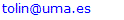Abstract

This experimental work deals with the influence of the angle of attack (AoA) and the chord based Reynolds number (Rec) on the lift and drag coefficients for a low-aspect-ratio NACA0012 airfoil. In addition, we provide novel general correlations for the minimum drag coefficient together with the ratio between the maximum lift and the minimum drag coefficient for different Reynolds numbers and several aspect ratios, after comparing our experimental data with other research works. This information is very useful for future validation of numerical simulations. Furthermore, we observe that the change in the aerodynamic characteristics are linked to the variations in the linear slope of the lift coefficient as function of AoA for any aspect ratio, thus finding a critical Reynolds number Rec=105 at which the slope saturates its value and the maximum of the polar curve changes its upward trend.

Keywords: Low Reynolds Number, Wing Aerodynamics, Aspect ratio, Wingtip Vortex

Cite this paper: S. Martínez-Aranda, A. L. García-González, L. Parras, J. F. Velázquez-Navarro, C. del Pino, Comparison of the Aerodynamic Characteristics of the NACA0012 Airfoil at Low-to-Moderate Reynolds Numbers for any Aspect Ratio, International Journal of Aerospace Sciences, Vol. 4 No. 1, 2016, pp. 1-8. doi: 10.5923/j.aerospace.20160401.01.

### 1. Introduction

Recent research in unmanned aerial vehicles (UAVs) deals with experimental measurements in which the wings present different aerodynamic characteristics as the chord Reynolds number or the aspect ratio are varied. It is also of great interest the aerodynamic performance regarding the maximum lift, lift curve slope, and polar curves . Typically, UAVs operate in the range between 50,000 and 150,000 chord Reynolds number. There is also a great interest to study 3D shape-change and optimization frameworks where the aspect ratio plays a significant role [2-3]. This is the motivation of our experimental study: to analyse the aerodynamic characteristics at low-to-moderate Reynolds numbers in order to compare our results with other works with different aspect ratios.
A wing profile is a surface that might be designed to provide lift force and the minimum drag. The relationship between both forces is determined by the wing cross section aerodynamic features . In finite wings at low Reynolds numbers, drag and lift coefficient variations are mainly due to three mechanisms: Wingtip vortex [5-9]; laminar boundary layer separation leading to the formation of a laminar separation bubble (LSB) and the subsequent turbulent separated shear layer [10-12] and finally the vortex shedding in the wake behind the wing [13-15]. Most of these numerical or experimental investigations were performed analysing specifically the flow behaviour. Hence, CD and CL coefficients involve a whole fluid-structure scenario, giving us an overview of the interaction between the wing and the flow that passes over it.
The NACA0012 airfoil has been extensively studied and its aerodynamic features are well known as well as the comparison between numerical and experiments by using Large Eddy Simulation . Abbot and von Doenhoff  presented a large experimental data summary for different 2D airfoils. The maximum CL observed by these authors was 1.1 - 1.6 for chord based Reynolds numbers ranging from 3·106 to 9·106, and with stall angles between 12º and 16º. For lower angles than stall, the lift coefficient increases linearly with a constant slope ΔCL/Δα. The minimum CD depends on the roughness of the wing surface. Two decades later, Sheldahl and Klimas  tested a 2D NACA0012 model for chord based Reynolds numbers between 3.6·105 ≤ Rec ≤ 7·105 and AoA in the range 0º < α< 180º. Their results showed a reduction of the maximum lift coefficient (CLmax = 0.9 - 1.0) and the stall angle (αstall = 10º - 12º) in comparison to the study of Abbot and von Doenhoff , though the slope of the lift curves as function of α for small AoA were in agreement. It was also observed by Sheldahl and Klimas  that the slope decreases for the AoA greater than 5º - 6º. Nevertheless, if the aspect ratio (AR) between the chord and the span of the wing decreases up to a certain limit value, three-dimensional effects on the wingtip dynamics play a key role. This modifies the expected aerodynamics performance of the wing and there is a meandering movement of the vortex core that is complex to identify [18, 19], though low frequencies have been measured in this random movement for the same NACA0012 airfoil studied here by means of flow visualizations and Proper Orthogonal Decomposition . Besides, the vortex core location has been adjusted while doing PIV measurements due to the presence of the vortex wandering . Actually, wingtip vortex on finite wings generates a lift coefficient reduction respect to the 2D infinite airfoil (called “downwash”), as well as the increment of the drag coefficient. This behaviour is even more significant if the aspect ratio reduces its value. Laitone  performed tests with a rectangular finite wing with a NACA0012 cross section profile, with AR=6, chord based Reynolds numbers below 7·104, and a free stream turbulence intensity 0.02% - 0.1%. These results showed a discontinuity at small AoA in the slope on the linear region of the lift curves for Rec = 2.07·104 and Rec = 4.21·104. This discontinuity decreases as the free stream velocity increases together with the smooth increment of the slope ΔCL\Δα. Likewise, the maximum lift coefficient for a constant Reynolds number decreases as the free stream turbulence intensity increases. Laitone also reported a slight reduction of the minimum drag coefficient C_Dmin (null AoA) as Rec increases. The CDmin evolution with Reynolds number has a curve fitting C_Dmin=0.35·Rec-0.25. Moreover, Nngo and Barlow  conducted measurements in a low turbulence wind tunnel of a rectangular NACA0012 (AR≈4) in order to study the mechanism that reduces the drag force induced by the wingtip vortex for Rec = 4.8·105. They obtained a value of CDmin = 0.048 for α = 0º, while CLmax is close 0.6 for the critical (stall) angle αstall = 11º. These values are reviewed to highlight how the aerodynamic characteristics of the NACA0012 airfoil strongly depends on the geometry (e.g. aspect ratio) and the presence of edge effects.
Finally, Mueller  measured experimentally drag and lift coefficients for different wing models in a wind tunnel. These models had semi-span aspect ratios (sAR) between 0.5 and 3 and they were machined using thin flat plates with rounded edges. The Reynolds numbers tested were in the range between 6·104 and 2·105, and AoA between -15º and +25º. Mueller found a direct relationship between the wing aspect ratio and the drag and lift polar profiles. Specifically, for a rectangular flat plate model and decreasing the sAR from 3 to 0.5, the lift coefficient is reduced by 50% in comparison to the rounded edge model. However, as the semi-aspect ratio is decreased, the linear region of CL is extended to greater values of α, as well as an increment of the stall angle. In other work , the same author reported a value of CLmax = 0.8 with a stall angle of 18º for the (flat plate) rectangular wing model with aspect ratio AR = 2, together with other experimental observation: an increase of the drag force due to the occurrence of edge effects, not moving from squared to rounded edges but due to wingtip vortex formation.
The outline of this manuscript is as follows. Details of the experimental setup are given in Section 2 including a brief description on aerodynamic coefficient computations. Results and discussions are shown in order to achieve a better understanding of the general curve fittings for several aspect ratios in Section 3, including an estimation of sources of experimental errors in different flow regimes. Finally, we draw the main findings in Section 4.

### 2. Experimental Arrangement

Experimental tests are performed in the Vehicle Aero-Hydrodynamics Laboratory (VAHL) at Málaga University, with a closed (return-flow) low-speed wind tunnel, which has a 4 m long closed test section, with one squared meter cross-section. The free-stream velocity ranging from U = 4 to 30 m/s. The turbulence level (Turbulence Intensity I [%]) is shown in Table 1. The calibration of the free-stream velocity was done by means of Laser Doppler Anemometry (LDA). The percentage of power %P supplied to the four wind tunnel fans (relative to their nominal power) is the control parameter. This parameter produces a wind velocity U with a given deviation δU∞ LDA. The values %P, U∞ LDA and δU∞ LDA are also shown in Table 1. We use four different free-stream velocities. The chord based Reynolds number Rec is defined as Rec = U·c/ν; ν being the temperature-dependent kinematic viscosity of the air. The blockage ratio is lower than 1\%, so this effect is negligible. The model is a solid rectangular wing NACA0012 airfoil. The wing model has a chord and a length of c=100 mm and l=200 mm, respectively, so the aspect ratio is AR = l/c = 2. The NACA0012 airfoil is made from an aluminium alloy with rounded wingtip. The maximum thickness is 12 mm and it is located at 30 mm from the profile leading edge [20, 21].
The wing is firmly fixed to a cylindrical base, also made of aluminium, allowing the coupling of the wing to a precision force sensor placed under the test section floor (see the sketch in Figure 1 and photographs in Figure 2). This digital device measures 3D forces and it is levelled taking into account the location of the Z-axis in the vertical position respect to the measurement section floor, in other words, Z-axis is parallel to the gravitational force. The X-Y force sensor plane is perpendicular to the longitudinal axis of the wing, the X-axis being parallel to U. The force sensor is coupled to an automatic rotation system which allows to vary the wing-base system orientation in the range of α = [-180º, 180º]. The rotation mechanism was previously calibrated to ensure its accuracy .
Experiments are carried out during T = 200 s, and for the AoA ranging from α = 0º to α = +35º constraining the rotation angle to 1 or 2 degrees increments, depending on the case.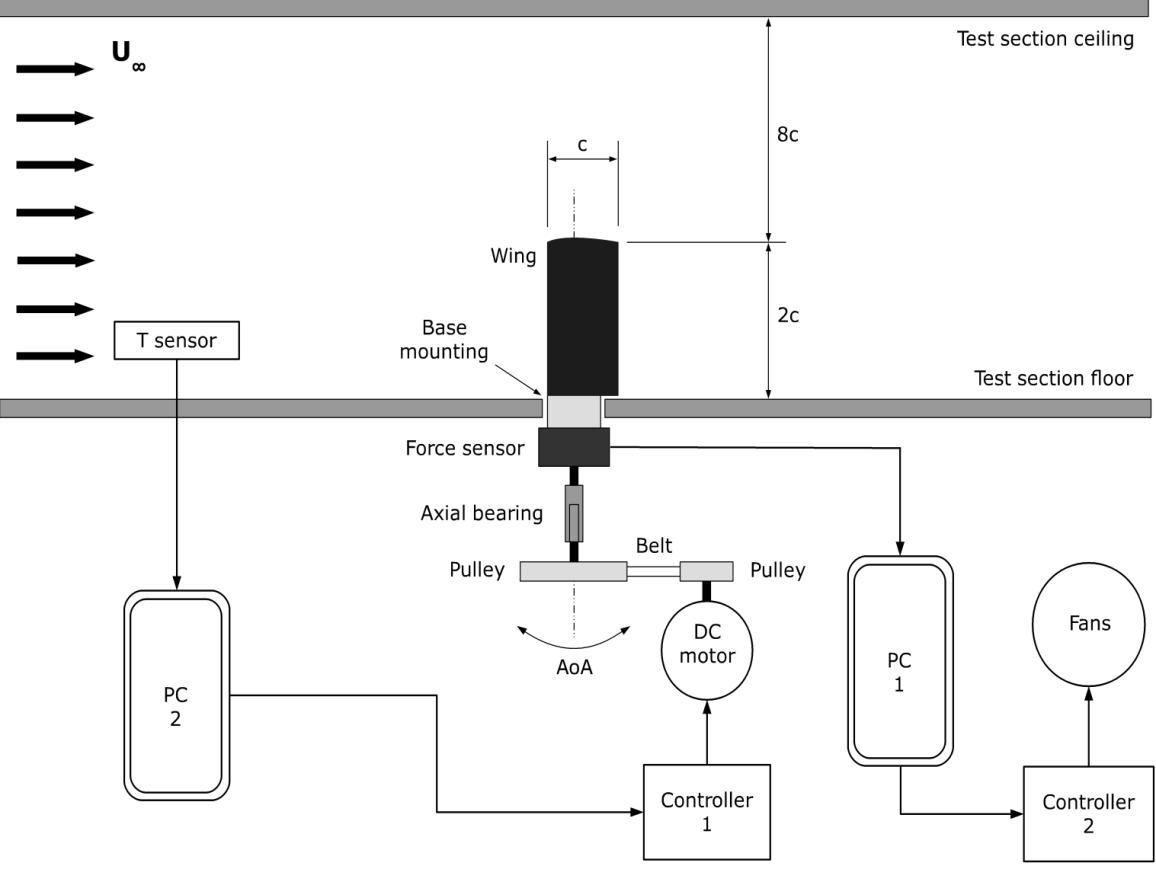Figure 1. Schematics of experimental set-up with the wing model mounted
 Table 1. Percentage of power, wind velocities and their variations, chord based Reynolds numbers and levels of turbulence intensity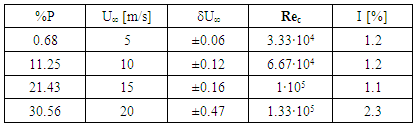We record forces in each orthogonal direction in the range 0 V ≤ Voutput ≤ 5 V, with a sampling frequency of fs = 250 points/s. The conversion factor between the force and the electrical output is k = 32N/5V. The rotation system is also calibrated in order to find the null angle where the lift force is equal to zero and the drag force presents minimum values.
This is done to find the angular position in a relative coordinate system (respect to the X-axis of the force sensor) at which the airfoil is aligned to the free-stream direction. Hence, a set of tests are conducted for the AoA between -10º ≤ α ≤ +10º (not shown) in order to detect the symmetry axis of the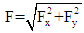as function of α, that corresponds to the angle where the force F presents its minimum value. Besides, and following an accurate experimental procedure , a set of experiments for different AoA are carried out without velocity in the tunnel in order to determine the force offsets (FX0,FY0) in the plane X-Y, and possible deviations in the Z axis measuring the weight of the wing. Therefore, the expressions for the different angles and forces are: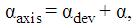(1)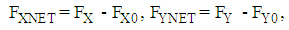(2)
Where α is the AoA, αdev is the angle between the X force sensor axis and the null AoA, and αaxis is the angle between the X force sensor axis and the drag direction. FX, FY correspond to raw data from the force sensor in the axis X and Y, respectively, and FXnet, FYnet are net forces in X and Y directions. Net forces are post-processed using Matlab® to obtain the lift and drag forces as follows: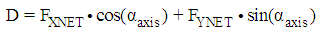(3)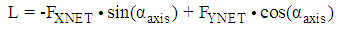(4)
D, L being the drag and lift forces on the wing model, which are used to compute the drag and lift non-dimensional coefficients. We calculate these coefficients taking into account the air density as function of temperature ρ(T). Tests are conducted continuously, acquiring the air temperature inside the tunnel for each experiment. Fluctuations in the air temperature are within 0.2 K for a single test. The equations to compute drag and lift coefficients are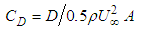(5)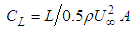(6)
ρ being the air density inside the wind tunnel, and A = l·c the aerodynamic area of the rectangular model (200 · 100 mm2). We repeat three times each experiment to obtain average values. The relaxation time between measurements at each AoA was large enough to avoid any kind of perturbation. Besides, the unsteady force was monitored at each AoA to ensure that the force reached a settled value.
A photograph of the experimental set up real is shown in Figure 2. The photograph above has been obtained from inside the tunnel, and shows the NACA 0012. The photograph below shows the digital force sensor coupled to an automatic rotation system which allows to vary the wing-base system orientation.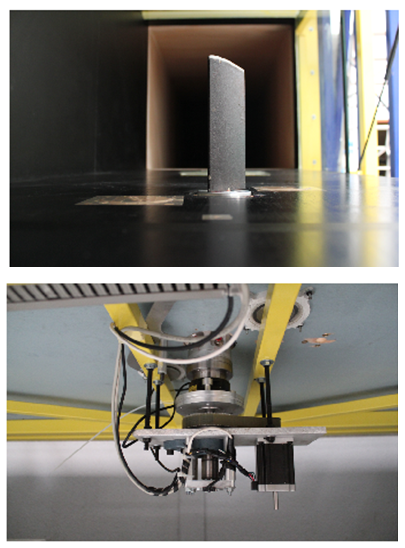Figure 2. Up: NACA 0012 profile inside the wind tunnel; Down: digital force sensor coupled to an automatic rotation system which allows to vary the wing-base system orientation in the range of α = [-180º, 180º]

### 3. Aerodynamic Characteristics: Results and Discussion

Drag coefficients as function of α are depicted in Figure 3. One can observe in the inset that our results also show a slight deviation in comparison to those obtained by Ngo . Besides, our values of CDmin are in agreement with those reported by Mueller  for a flat wing with the same aspect ratio and Reynolds numbers. However, our results differ from those given by Sheldahl  for a bidimensional NACA0012 airfoil. The main cause of this discrepancy is the appearance of effects in the trailing edge, e.g. wingtip vortex formation, which dominate the wing aerodynamic performance due to the low-aspect-ratio of the model, AR = 2. In addition, the tip vortex constrains the laminar separation bubble at the leading edge. This effect was reported in Yen . For this reason, the drag coefficient induced by three-dimensional effects at null AoA is approximately five times greater than the value of CDmin for a 2D profile measured by Sheldahl .
Regarding the ratio between drag coefficient and AoA, ΔCD/Δα, we observe an increment of its value up to the stall angle, so that the wing loses its aerodynamic effectiveness. Different slopes are found at each Reynolds number, increasing the values of the slope with the AoA up to the stall angle. These ratios are of great interest in the case of the lift coefficient, as it will be discussed below.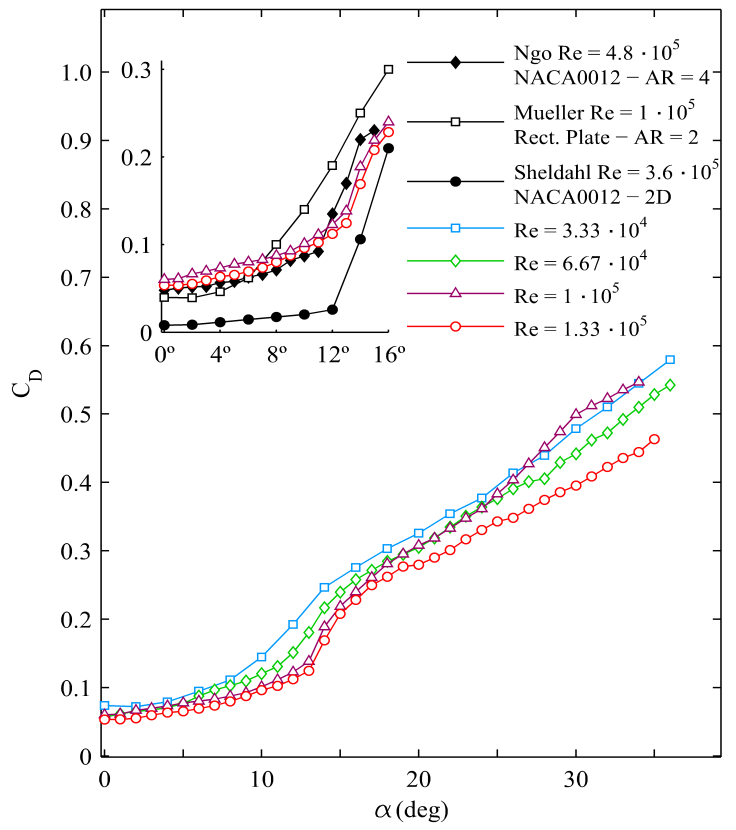Figure 3. CD vs α for all Reynolds numbers tested. The inset represents a zoom of CD values for low angles of attack and high Reynolds numbers, together with those reported by Ngo and Barlow , Mueller and Torres  and Sheldahl and Klimas 
The lift coefficient versus AoA is shown in Figure 4, and it presents a reduction of 40% in the maximum lift coefficient CLmax in comparison to the values measured by Sheldahl  for a 2D model. The maximum lift coefficient CLmax has values between 0.52 and 0.61 for any value of the Reynolds numbers, and the stall angles stall appear between 12 and 14 degrees. These values show a slight deviation compared to those published by Ngo  with AR = 4. As expected, our stall angles are slightly greater than Ngo's results due to the aspect ratio reduction (AR = 2 in our case). It is worth mentioning that the values of CL are lower than those obtained by Mueller  for a flat plate with the same aspect ratio and Reynolds numbers. Furthermore, βL defines the ratio between the lift coefficient and the AoA, ΔCL/Δα, see the inset in Figure 4.
In this work, we suggest a classification of the wing aerodynamics defining βL as function of AoA. We observe two different slopes, so we split βL into two, depending on the value of α: βL1 (for null AoA up to α1 ≈ 3º) and βL2 (from α1 till the stall angle). βL1 and βL2 for the two highest Reynolds numbers are plotted in the inset of Figure 4 together with the results from Prandtl's lifting line theory for symmetric, rectangular wing with no twist and finite span, CL ≈ mα, with m = 2π/[1 + 1/(sAR)] . The slopes βL1 for highest Reynolds numbers are 0.059 deg-1 and 0.063 deg-1 for Rec = 1·105 and Rec = 1.33·105, respectively. These slopes at the origin are far from those reported by Sheldahl  for a 2D model and Rec of the same order of magnitude (βL1 = 0.11 deg-1). However, these data are practically in agreement with those reported by Mueller  for small AoA and for a flat plate with AR = 2 (βL1 = 0.05 deg-1) and with those obtained by Ngo  for a NACA0012 airfoil with AR = 4 (βL1 = 0.075 deg-1).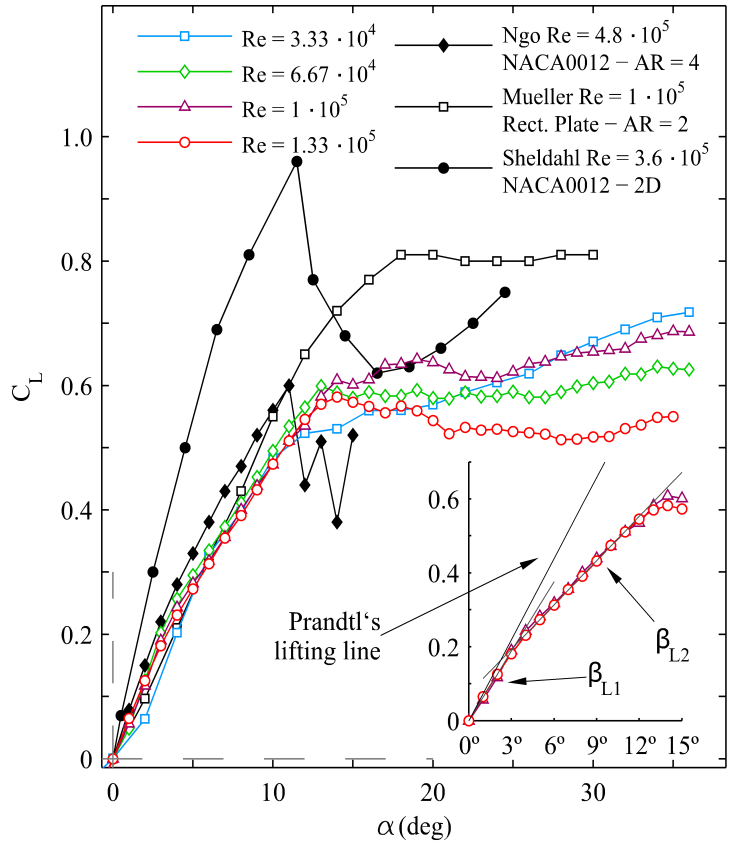Figure 4. CL vs α for all Reynolds numbers together with those reported by Ngo and Barlow , Mueller and Torres  and Sheldahl and Klimas . The inset shows detail of βL1 and βL2 for the two highest Reynolds numbers in comparison to the result from Prandtl’s lifting line theory for symmetric, rectangular wing with no twist and finite span 
For the two lowest Reynolds numbers, the linear region of the lift curve (before stall) has a discontinuity at small AoA, see Figure 5. The values of βL1 correspond to the slope before the discontinuity appears, so the values are 0.032 deg-1 and 0.048 deg-1 for Rec = 3.33·104 and 6.67·104, respectively. On the one hand, these slopes show an excellent agreement with those reported by Laitone  for similar Rec, in a NACA0012 wing with AR = 6 and AoA close to zero. On the other hand, the lift coefficients curves show a similar behaviour to those achieved by Laitone: as the free stream velocity increases, the discontinuity of slope βL1 is smoothly reduced, so the final tendency is a constant slope at small AoA (0º ≤ α ≤ 3º). Once parameters βL1, βL2 are defined and characterized, we will discuss later our curve fitting as function of Rec and any value of the aspect ratio AR. We first analyse the minimum value of CD.
The minimum drag coefficient decreases as Rec increases. The experimental points depicted in Figure 6 (a) are adjusted using the following equation CDmin = 0.55·Rec-0.21. Laitone  obtained the equation CDmin = 0.35·Rec-0.25 to correlate the minimum drag coefficient for 2·104 ≤ Rec ≤ 7·104. The main cause of this difference, especially in the range of CDmin, are the different aspect ratios used in both experiments and the different range of Reynolds numbers. Nevertheless, we suggest a general correlation using the following expression for any value of AR: CDmin·AR = 2·Rec-0.25. This curve fitting is plotted in Figure 6 (b). There is a reasonable good agreement for the data reported by other authors [22, 14, 17 and 9] for different AR and Rec. We present only a disagreement with those results reported by Ngo  and Yen . The discrepancy may be explained in terms of edge effects, surface roughness or rounded shape, the minimum offset used in the force sensor or the turbulence intensity in the wind tunnel. Nevertheless, it is worth mentioning that four different experimental arrangements give data that collapse in one curve fitting.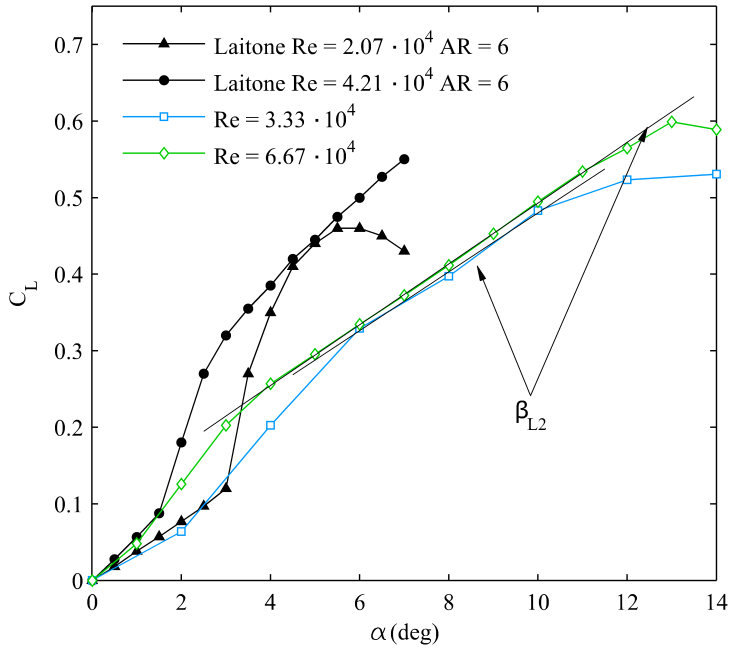Figure 5. CL vs αfor Rec = 3.33·104 and Rec = 6.67·104 with AoA values lower than the stall angle (AR = 2), together with Laitone’s data  (AR = 6, I = 0.02%)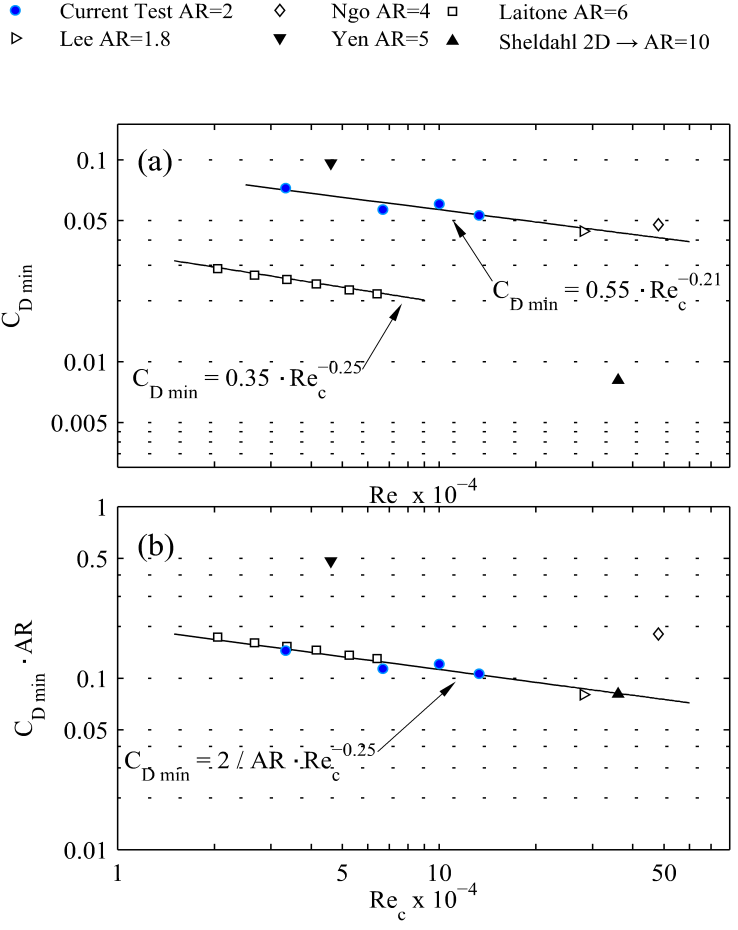Figure 6. (a) CDmin vs Rec for 3.33·104≤Rec≤1.33·105 and AR = 2 compared with the results of Ngo and Barlow , Yen and Huang , Laitone , Lee and Pereira  and Sheldahl and Klimas . (b) CDmin · AR vs Rec for the universal NACA 0012 wings fitting law CDmin = 2/AR·Rec−0.25
Other general correlations for βL1 and βL2 as function of Rec for NACA0012 finite wings and any AR are shown in Figure 7. For Rec lower than 1·105 the βL1 slope is equal to βL1·AR-0.5=0.00012·Rec0.51, and βL1·AR-0.5 ≈ 0.042 for Rec greater than 1·105 whilst βL2·AR-0.5 = 0.056·Rec-0.07 for any value of Rec. In the case of our curve fitting βL1·AR-0.5, there is an excellent agreement for all the cases reported. The function βL1·AR-0.5 presents a linear dependence on the Reynolds number up to Rec ≤ 1·105, but for greater values the function βL1·AR-0.5 remains almost constant. It is clear that there is a critical value of the chord based Reynolds number at Rec = 1·105, where βL1 finds a saturation value which strongly depends on the aspect ratio. Regarding βL2·AR-0.5, there is a reasonable good agreement with all the data reported for several AR.
Finally, the polar curve CL/CD against AoA is plotted in Figure 8 together with CD as function of CL. The maximum value CL/CD increases with Rec. However, once Rec is greater than 1·105, there is a variation in the upward trend, so that the maximum value decreases with Rec instead of increasing its value. Obviously, this critical Reynolds number tends to coincide with the value at which the ratio βL1 saturates, giving validity and reliability to the curve fitting presented in Figure 9 (a). The maximum efficiency is found in the polar curve between 10º and 12º, depending on Rec. In addition, CLmax/CDmin is plotted against Rec in Figure 9 (a) together with those data provided by other authors [23, 25, 22, 9 and 17]. Again, we suggest a general correlation for NACA0012 airfoils and any value of the aspect ratio, AR, the final expression being CLmax/CDmin = 0.27·AR·Rec0.25. There is a reasonable good agreement, so most data collapse in one curve fitting, see Figure 9 (b).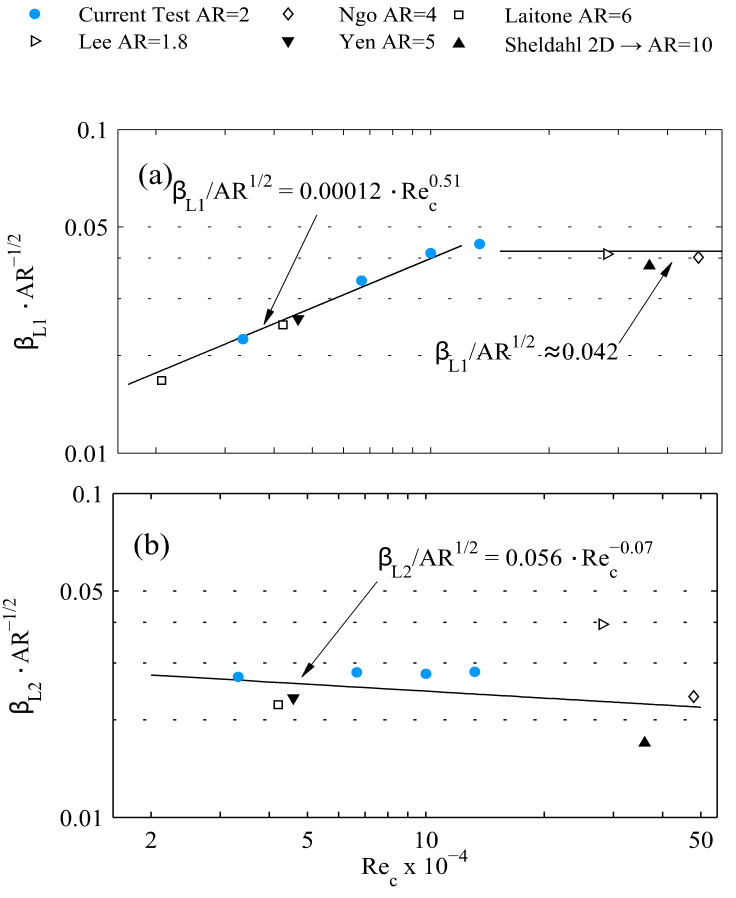Figure 7. Curve fitting for βL1 ·AR-0.5 vs Rec (a) and curve fitting for βL2·AR-0.5 vs Rec (a) for 3.33·104 ≤Rec ≤1.33·105 and AR = 2 (b) together with those results reported by Ngo and Barlow , Yen and Huang , Laitone  and Lee and Pereira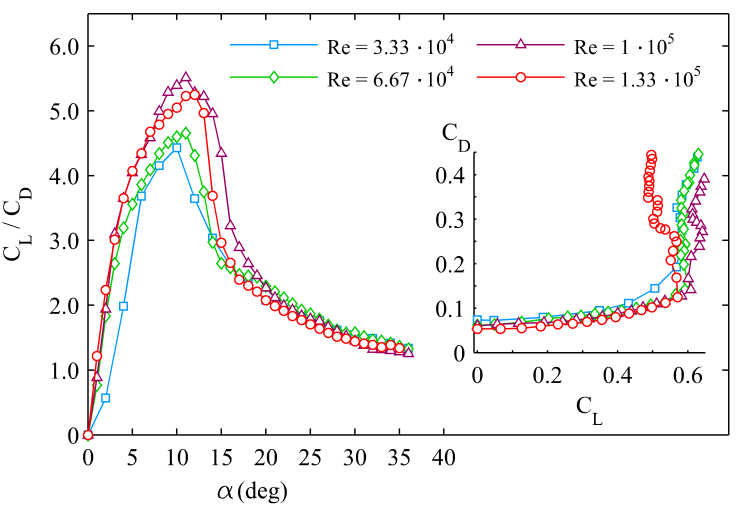Figure 8. Lift-Drag ratio CL/CD vs angle of attack α. The inset shows CD vs CL for all Reynolds numbers tested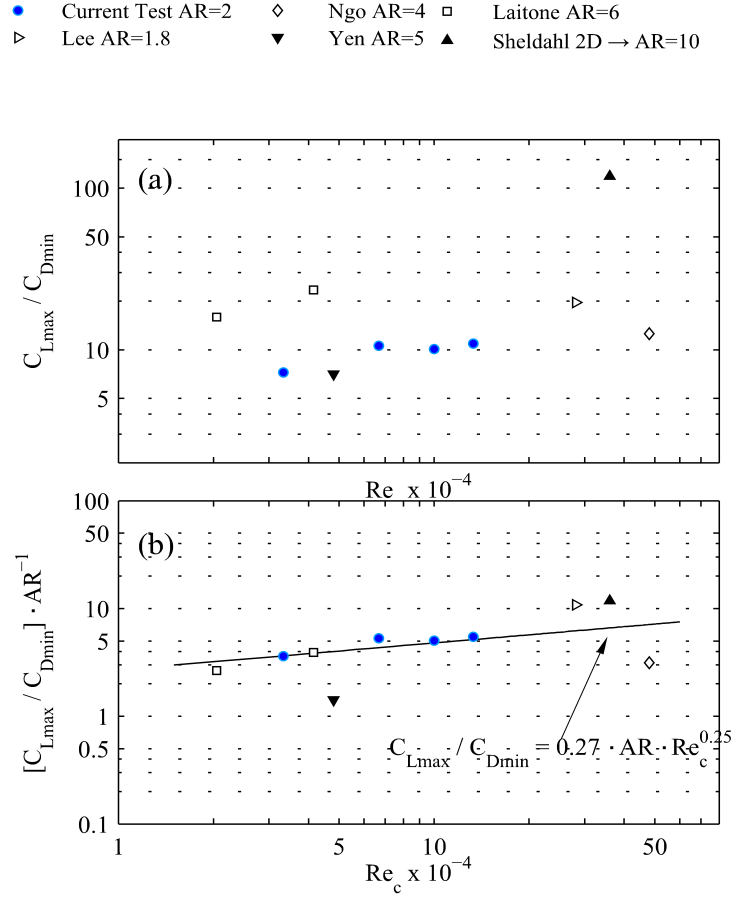Figure 9. (a) CLmax/CDmin vs Rec for 3.33·104 ≤ Rec ≤ 1.33·105 and AR = 2 compared with the results of Ngo and Barlow , Yen and Huang , Laitone , Lee and Pereira  and Sheldahl and Klimas . (b) CLmax/CDmin·AR−1 versus Rec for the fitting law CLmax/CDmin=0.27·AR·Rec0.25

### 4. Conclusions

The precise dynamic response of low-aspect-ratio NACA0012 airfoil (aspect ratio, AR = 2) has been characterized by means of a digital force sensor at low-to-moderate Reynolds numbers. We compute drag and lift coefficients through the temporal evolution of force measurements. We show in this experimental study that wingtip vortex generation and low-aspect-ratio are the main causes of the lift force reduction in comparison to the NACA0012 (bidimensional) infinite profile, being this reduction almost 40% for any value of the Reynolds number. In addition, the edge effect generates an induced drag force which provides a value six times greater than the (bidimensional) infinite profile. Finally, and regarding the aerodynamic characteristics, the critical (stall) angle, αstall, takes place at 12 degrees approximately, and this angle increases with the Reynolds number slightly.
On the other hand, the relation between CDmin and Rec has been experimentally adjusted and verified with previous studies for any AR by the expression CDmin = 2/AR·Rec-0.25. Besides, we pay our attention on the lift coefficient, so we define the ratio between the increment of lift coefficient and the angle of attack, ΔCL/Δα, namely βL1 for 0º ≤ α ≤ 3º and βL2 for 0º ≤ α ≤ αstall. Our main finding of this experimental study is the general curve fittings for βL1 and βL2, and any value of AR. We find that βL1· AR-0.5 strongly depends on Rec up to Rec ≈ 1·105 L1·AR-0.5=0.00012·Rec0.51), being βL1·AR-0.5 almost constant for Rec greater than 1·105L1·AR-0.5 ≈ 0.042). On the other hand, βL2·AR-0.5 is also constant for all Rec considered in this work (βL2·AR-0.5 = 0.056·Rec-0.07). In addition, we propose a general correlation for the relation CLmax/CDmin and any AR: CLmax/CDmin = 0.27·AR·Rec0.25.
Finally, we do observe that the critical chord based Reynolds number Rec ≈ 1·105 at which βL1 saturates its value is connected to the change of the upward trend in the maximum value of the polar curve. More effort must be done regarding forced vibration response. This is a work in progress.

### ACKNOWLEDGEMENTS

This research has been supported by Proyecto Junta de Excelencia de la Junta de Andalucía, Grant number P11-TEP-7776.

### References

  Ananda, G. K., Sukumar, P. P., Selig, M. S. 2015. Measured aerodynamic characteristics of wings at low Reynolds. Aerospace Science and Technology, 42, 392-406.  Boulkeraa, T., Ghenaiet, A., Mendez, S. and Mohammadi, B. 2014. A numerical optimization chain combining computational fluid dynamics and surrogate analysis for the aerodynamic design of airfoils. Proc IMechE Part G: Journal of Aerospace Engineering, 228 (11), 1964-1981.  Fincham, J. H. S., Friswell, M. I. (2015). Aerodynamic optimization of a camber morphing aerofoil. Aerospace Science and Technology,43, 245-255.  Abbott, I. H., von Doenhoff, A. E. Theory of wing sections. Dover Publications, Inc., New York. 1959.  Mueller, T. J. Aerodynamics measurement at low reynolds numbers for fixed wing micro-air vehicles. Hessert Centre for Aerospacial Research, Department of Aerospace and Mechanical Engineering, University of Notre Dame, Indiana (USA). 1999.  Mueller, T. J. and Torres, G. E. Aerodynamics of low aspect ratio wings at low reynolds numbers with application to micro-air vehicles design an optimization. Hessert Centre for Aerospacial Research, Department of Aerospace and Mechanical Engineering. 2001.  Karakus, C., Akilli, H. and Sahin, B. 2008. Formation, structure, and development of near-field wing tip vortices Proc IMechE Part G: Journal of Aerospace Engineering, 222 (1), 13-22  Lee, T., Su, Y. Y. 2012. Wing tip vortex control via the use of a reverse half-delta wing. Experiments in Fluids, 52, 1593-1609.  Lee, T. and Pereira, J. 2013. Modification of static-wing tip vortex via a slender half-delta wing. Journal of Fluids and Structures, 43, 1-14.  Gad-el Hak, M. 1990. Control of low-speed airfoil aerodynamics. AIAA Journal, 28 (9), 1537-1552.  Mueller, T. J. 1985. Low Reynolds number vehicles. AGARD-AG-288.  Huang, R. F., Lee, H. W. 1999. Effects of freestream turbulence on wing-surface flow and aero- dynamic performance. Journal of Aircraft, 36 (9), 965-972.  Huang, R. F., Lin, C. 1995. Vortex shedding and shear-layer instability of wing at low-Reynolds numbers. AIAA Journal, 33 (8), 1398-1403.  Lee, H.W., Huang, R. F. 1998. Frequency selection ofwake flowbehing aNACA0012 wing. Journal of Marine Science and Technology, 6 (1), 29-37.  Huang, R. F. and Lee, H.W. 2000. Turbulence effects on frequency characteristics of unsteady motions in wake of wing. AIAA Journal, 38 (1), 85-93.  Cuerno-Rejado, C., López-Martínez, G., Escudero-Arahuetes, J. L. and López-Díez, J. 2001. Experimental aerodynamic characteristics of NACA 0012 airfoils with simulated glaze and rime ice. Proc IMechE Part G: Journal of Aerospace Engineering, 215 (1), 229-240.  Sheldahl, R. E. and Klimas, P. C. 1981. Aerodynamics characteristics of seven symmetrical airfoil sections through 180 degree angle attack for use in aerodynamics analysis of vertical axis wind turbines. Sandia National Laboratories, Albuquerque (USA), SAND80-2114.  Beresh, S. J., Henfling, J. F., Spillers, R. W. 2010. Meander of a fin trailing vortex and the origin of its turbulence. Experiments in Fluids, 49, 599-611.  Mula, S. M., Stephenson, J. H., Tinney, C. E., Sirohi, J. 2013. Dynamical characteristics of the tip vortex from a four-bladed rotor in hover. Experiments in Fluids, 54 (1600).  del Pino, C., Lopez-Alonso, J. M., Parras, L., Fernandez-Feria, R. 2011. Dynamics of the wing-tip vortex in the near field of a NACA 0012 airfoil. The Aeronautical Journal, 115 (1166).  del Pino, C., Parras, L., Felli, M., Fernandez-Feria, R. 2011. Structure of trailing vortices: Comparison between particle image velocimetry measurements and theoretical models. Physics of Fluids, 23, 113602.  Laitone, E. V. 1997. Wind tunnel test of wings al Reynolds number below 70000. Experiments in Fluids, 23, 405-409.  Ngo, H. T. and Barlow, L. E. Lifting surface with active variable tip member and method for influencing lifting surface behavior therewith. United State Patent No. US 6.394.397 B1. 2002.  Fedoul, F., Parras, L., del Pino, C., Fernandez-Feria, R. 2014. Experimental study of the aero- dynamic characteristics of a low-aspectratio flat plate array in a configuration of interest for a tidal energy converter. Journal of Fluids and Structures, 48, 487-496.  Yen, S. C. and Huang, L. C. 2009. Flow patterns and aerodynamics performance of unswept and swept-back wings. Journal of Fluids Engineering, 131 (11).